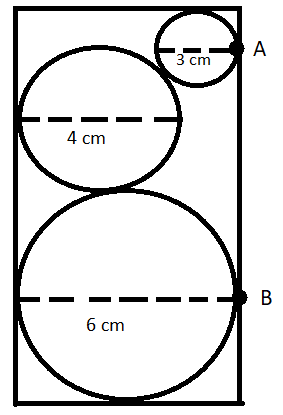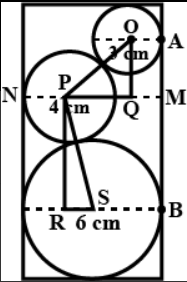QUESTION

# Using the given figure, find the length of AB.(a) 8 cm(b) 4.5 cm(c) 7.35 cm(d) 7.30 cm

Hint: First, find the radii of the circles. Redraw the diagram to understand things better. Apply Pythagoras theorem on the two right angles formed in the redrawn figure to find the length of PR and OQ which are equal to MB and AM, respectively. Add these to find the final answer.

In this question, we have to find the length of the segment AB using the given figure.
We are given the length of the diameters of the three circles. We will find the radii of these circles.

We know that radius is half of the diameter. Using this we will get the following:
Radius of 3 cm diameter circle = 1.5 cm
Radius of 4 cm diameter circle = 2 cm
Radius of 6 cm diameter circle = 3 cm

We will now redraw the given diagram as:Finding the length of MB:
PS = 2 + 3 = 5 cm.
SR = 3 - 2 = 1 cm .

Also, triangle PRS is a right angled triangle. So we will use the Pythagoras theorem on triangle PRS.
$P{{R}^{2}}+S{{R}^{2}}=P{{S}^{2}}$
$P{{R}^{2}}+{{1}^{2}}={{5}^{2}}$
$P{{R}^{2}}=24$
$PR=\sqrt{24}=2\sqrt{6}$ cm.

Also, PR = MB
So, the length of MB is $2\sqrt{6}$ cm. …(1)

Finding the length of AM :
OP = 1.5 + 2 = 3.5 cm
PQ = MN - PN - MQ = 6 - 2 - 1.5 = 2.5 cm

Triangle OQP is a right angled triangle. So we will use the Pythagoras theorem on triangle OQP.
$O{{Q}^{2}}+P{{Q}^{2}}=O{{P}^{2}}$
$O{{Q}^{2}}+{{2.5}^{2}}={{3.5}^{2}}$
$O{{Q}^{2}}=6$
$OQ=\sqrt{6}$ cm

Also, OQ = AM.
So, the length of AM = $\sqrt{6}$ cm. …(2)
Now, we can see that AB = AM + MB
So, from (1) and (2), we will get the following:
$AB=2\sqrt{6}+\sqrt{6}$
$AB=3\sqrt{6}=7.35$ cm
Hence, option (c) is correct.

Note: In this question, it is very important to redraw the given diagram to understand things better. Also, it is important to know that if we join the centres of two tangential circles, the line joining the centre has the length equal to the sum of the radii of the circles.